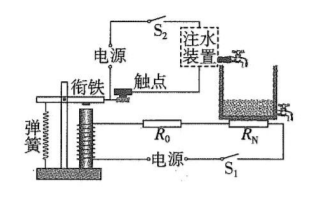$\text{A.}$ 水位上升时, 电磁铁磁性变弱 $\text{B.}$ 水位上升时, 通过 $R_0$ 电流变小 $\text{C.}$ 水位下降时, $R_0$ 的电功率变大 $\text{D.}$ 水位下降时, $R_{\mathrm{N}}$ 的阻值会变大

D

#### 解析：

CD. 水位下降时, 需要注水, 触点接触, 电磁铁磁性减弱, 故 $R_{\mathrm{N}}$ 的阻值会变大, 电流变小, 根据 $P=I^2 R$ 可 知, $R_0$ 的电功率变小, 故 $\mathrm{C}$ 错误, D 正确。

①点击 收藏 此题， 扫码注册关注公众号接收信息推送（一月四份试卷,中1+高2+研1）
② 程序开发、服务器资源都需要大量的钱，如果你感觉本站好或者受到到帮助，欢迎赞助本站,赞助方式：微信/支付宝转账到 18155261033

 ①此题难易度如何 ②此题推荐度如何 确定